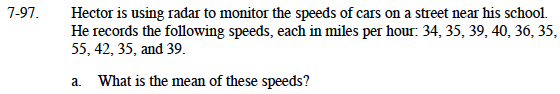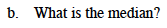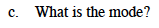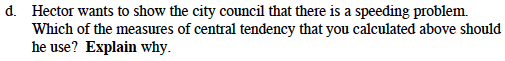### Home > MC1 > Chapter 7 > Lesson 7.2.5 > Problem7-97

7-97.To calculate the mean, find the sum of the data and divide it by the number of speeds.In the numerically-arranged data listed below, which number falls in the middle?
If there are two numbers in the middle, you need to find the mean of them.
34, 35, 35, 35, 36, 39, 39, 40, 42, 55Which speed is repeated most in the data list?Which of the answers you calculated best proves that there is a problem with speeding?

Since the mean is the highest speed of the measures of central tendency, it is most effective for proving that speed is an issue.